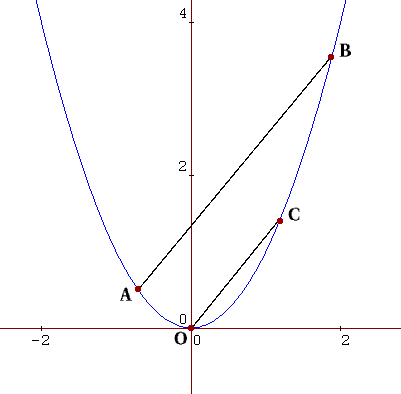#### You may also like### Power Up

Show without recourse to any calculating aid that 7^{1/2} + 7^{1/3} + 7^{1/4} < 7 and 4^{1/2} + 4^{1/3} + 4^{1/4} > 4 . Sketch the graph of f(x) = x^{1/2} + x^{1/3} + x^{1/4} -x### Snookered

In a snooker game the brown ball was on the lip of the pocket but it could not be hit directly as the black ball was in the way. How could it be potted by playing the white ball off a cushion?### Climbing

Sketch the graphs of y = sin x and y = tan x and some straight lines. Prove some inequalities.

# Parabella

##### Age 16 to 18Challenge Level

No the title is not a spelling mistake! This is a beautiful result involving a parabola and parallels. Take any two points $A$ and $B$ on the parabola $y=x^2$.

Draw the line $OC$ through the origin, parallel to $AB$ , cutting the parabola at $C$ .

Let $A=(a,a^2)$, $B= (b, b^2 )$, and $C= (c, c^2 )$. Prove that $a+b=c$.Imagine drawing another parallel line $DE$, where $D$ and $E$ are two other points on the parabola. Extend the ideas of the previous result to prove that the midpoints of each of the three parallel lines lie on a straight line.